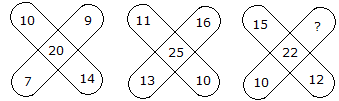# Verbal Reasoning - Character Puzzles - Discussion

### Discussion :: Character Puzzles - Character Puzzles 1 (Q.No.47)

47.

Which one will replace the question mark ?[A]. 6 [B]. 7 [C]. 8 [D]. 9

Explanation:

(10 + 9 + 14 + 7)/2 = 20

and (11 + 16 + 10 + 13)/2 = 25

Therefore, (15 + ? + 12 + 10)/2 = 22

Hence 37 + ? = 44

? = 44 - 37

? = 7.

 Ashifa said: (Jan 28, 2021) How 44 comes? Explain me.

 Proshanta Kumar Roy said: (Mar 1, 2021) How 44 comes? Please explain.

 Betty said: (Mar 21, 2021) (15+10+12+?)/2 =22. (37+?)/2 = 22, 37+? = 22x2 = 44, ? = 44 - 37 = 7.

 Dhaval said: (Jul 14, 2021) Why divided by 2? Explain.